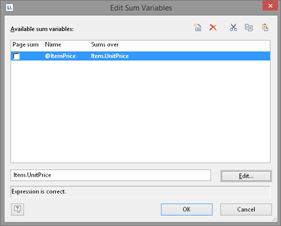+1 800 256 3608 (toll-free in North America) or +49 7531 90 60 10| service@combit.com# Sum variable across groups

Hi,
I have problem with sum variables across the tables and group lines.

I have report container with table_1 and nested table_2. The report container looks like this:

table_2:Data line - Here “some_field” is shown
table_2:Footer - Sum of “some_field” - Sum(some_field)

table_1:Group Footer 1 - Show @Sum01

Then I have @Sum01 sum variable with content “some_field” (so sum of field from the table_2).

Now, the @Sum01 in “table_1:Group Footer 1” does show wrong value. It contains 1 value of “some_field” more.
That is if “some_field” is always 1 and there are 4 data lines in “table_2:Data line” then @Sum01 is 5, instead of 4. It looks like the @Sum01 does accumulate “some_field” also in the “table_2:Footer”.

Can I somehow enforce that the value of the sum variable is updated ONLY in the data lines?

Thank you

You must tell the system for what table to use.

Instead of some_field, you should use:

Cond(CurrentContainerItem = “Table2”, SomeField, 0)

I hope that helps
Marco

1 Like

Hi,
thanks for the reply. Yes, it works(so when the footer is processed, the LL.CurrentContainerItem isn’t set? Or how does it works?)

But I’m bit confused why this expression is needed. It looks like that if the L&L is processing the table_2:Footer then the sum variable is still updated, which is counter-intuitive. I had impression that the sum variables are updated only if the new values were provided to the L&L.

Maybe this article can help?

1 Like

Thanks for the reply. It helpedMaybe, this text should be added to the L&L documentation also?

It kind of is in chapter 7.3 “Sum Variables” of the designer manual (and in the corresponding online help topic). However I don’t expect anyone to know each and every chapter of the documentation - we should think of ways to make this more intuitive and obvious. As always, thanks for reaching out, Jozef.

Yes, I’ve looked at this chapter, but there is no in depth explanation as in KB. Maybe there is in a newer doc? (I’m using L&L 22).

In any way, the problem is solved, thanks for helping1 Like

For future reference - this is what it reads:

## Sum Variables

Sum variables offer another way of creating totals and counters and work fundamentally across tables.

They are therefore a good choice whenever you want to create totals across different table hierarchies.

In all other cases, we recommend the use of the aggregate functions Sum(), Count() and CountIf() for totals and counters. Aggregate functions are always table-specific. You can even produce statistical analyses directly with aggregate functions e.g. Median(), Variance(), StdDeviation(). You will find a list of all functions in the “Aggregate functions” function category in the formula wizard.

Sum variables can be used to create totals over data sets, e.g. to add up the “Item.UnitPrice” fields in a table in order to calculate the total price. Such totals are permitted for all numeric variables or for expressions that return a numeric value as the result.

But sum variables are also a convenient way of defining a counter which can be updated accordingly for each data record that is printed.

You can create a total across all data sets of a printed page (page totals) or across the entire project (grand totals).

You can use the global replace function (Ctrl+H) to rename sum variables later.Figure 7.23: Totaling with sum variables.

Proceed as follows to define the variables:

Choose Project > Sum Variables or the “Edit sum variables” button in the formula wizard.

In the dialog that follows, create a new sum variable via the “Insert a new variable” button.

An input dialog appears where you can enter a description of the new variable. Give the variable a meaningful name, the “@” character will be added automatically as a prefix.

Click the “Edit” button to open the formula wizard and assign a field or an expression to the new sum variable.

For example, select the numeric field “Item.UnitPrice” if you want to add up the “Item.UnitPrice” column.

You can, however, also perform aggregations with complex expressions provided that the result is a numeric value. For instance, you can add up the gross price from the net price and the VAT. Enter the following expression, for example, in the “Sums over” field:

Item.UnitPrice+ Item.UnitPrice* (Item.VAT/100)

If you don’t want to add up any values but merely want to create a counter or a numeration, the definition is simple: In the “Sums over” field, simply enter the value that is to be added to the existing counter.

The simplest case is a consecutive number that is increased by 1 for each data record. Just enter the value “1”.

If you define tables in table columns, you must specify the respective table name (e.g.‘MainTable’) for the counter, otherwise the data records of the “Subtable” will also be counted.

Cond(LL.CurrentContainerItem =“MainTable”,1,0)

The “Page sum” checkbox lets you specify whether the totals are to be set to 0 at the end of a page. In this way, you can define page totals and counters.

Once you have defined which sums are to be stored in which sum variables, you can use these sum variables in your objects. In the formula wizard, you will find the sum variables at the end of the variable list in the “Sum variables” folder. In the tool window “Variables-/Field-List”, the sum variables can be directly edited by double clicking and via a context menu.